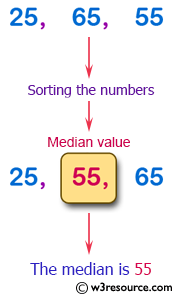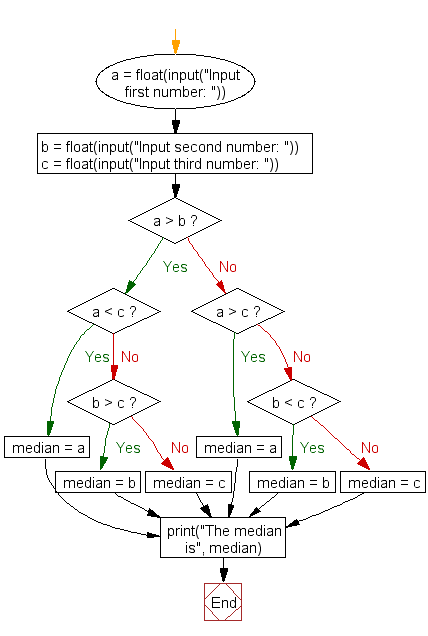﻿ Python Exercise: Find the median of three values - w3resource# Python Exercise: Find the median of three values

## Python Conditional: Exercise - 40 with Solution

Write a Python program to find the median of three values.

Pictorial Presentation:Sample Solution:

Python Code:

``````a = float(input("Input first number: "))
b = float(input("Input second number: "))
c = float(input("Input third number: "))
if a > b:
if a < c:
median = a
elif b > c:
median = b
else:
median = c
else:
if a > c:
median = a
elif b < c:
median = b
else:
median = c

print("The median is", median)
```
```

Sample Output:

```Input first number: 25
Input second number: 55
Input third number: 65
The median is 55.0
```

Flowchart :## Visualize Python code execution:

The following tool visualize what the computer is doing step-by-step as it executes the said program:

Python Code Editor:

Have another way to solve this solution? Contribute your code (and comments) through Disqus.

What is the difficulty level of this exercise?

Test your Python skills with w3resource's quiz

﻿

## Python: Tips of the Day

Function argument unpacking in Python.

Example:

```def tips_func(x, y, z):
print(x, y, z)

tuple_val = (2, 0, 2)
dict_val = {'x': 3, 'y': 2, 'z': 1}
tips_func(*tuple_val)
tips_func(**dict_val)
```

Output:

```2 0 2
3 2 1
```